# 4. (a) Show that mi = F - mw x W x D) - 2mw xi...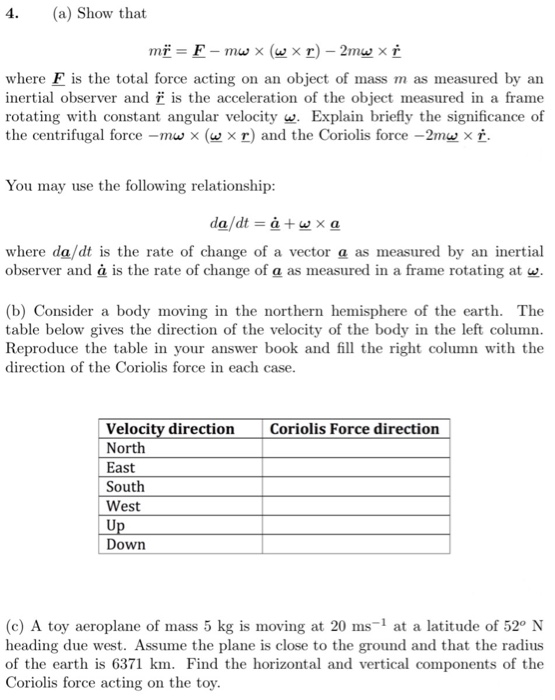4. (a) Show that mi = F - mw x W x D) - 2mw xi where F is the total force acting on an object of mass m as measured by an inertial observer and ï is the acceleration of the object measured in a frame rotating with constant angular velocity w. Explain briefly the significance of the centrifugal force –mw (w xr) and the Coriolis force -2mw xi. You may use the following relationship: da/dt = a +w xa where da/dt is the rate of change of a vector a as measured by an inertial observer and á is the rate of change of a as measured in a frame rotating at w. (b) Consider a body moving in the northern hemisphere of the earth. The table below gives the direction of the velocity of the body in the left column. Reproduce the table in your answer book and fill the right column with the direction of the Coriolis force in each case. Coriolis Force direction Velocity direction North East South West Up Down (c) A toy aeroplane of mass 5 kg is moving at 20 ms-1 at a latitude of 52° N heading due west. Assume the plane is close to the ground and that the radius of the earth is 6371 km. Find the horizontal and vertical components of the Coriolis force acting on the toy.

I have solved all the parts. If any confusion, please comment. Thank you.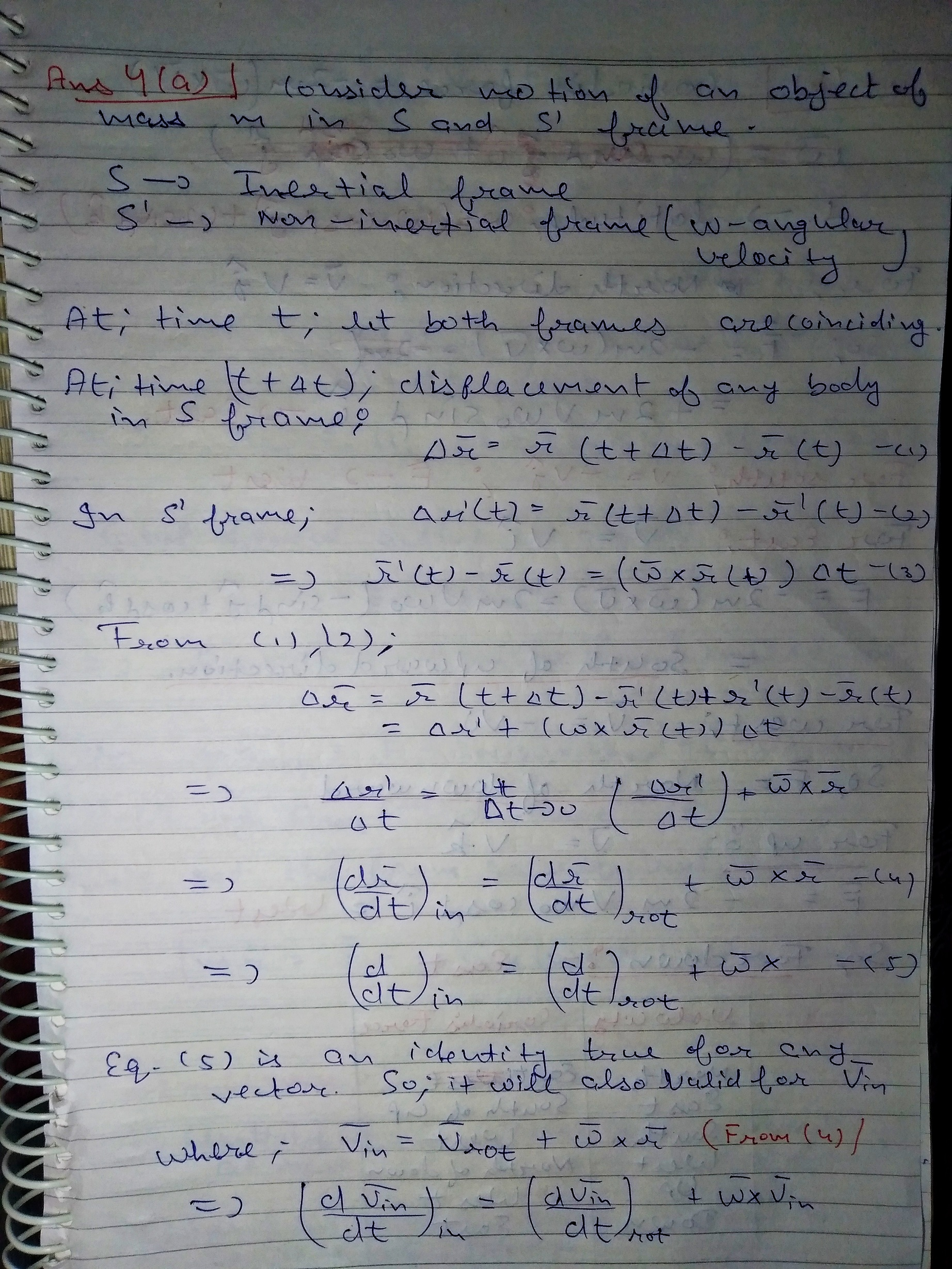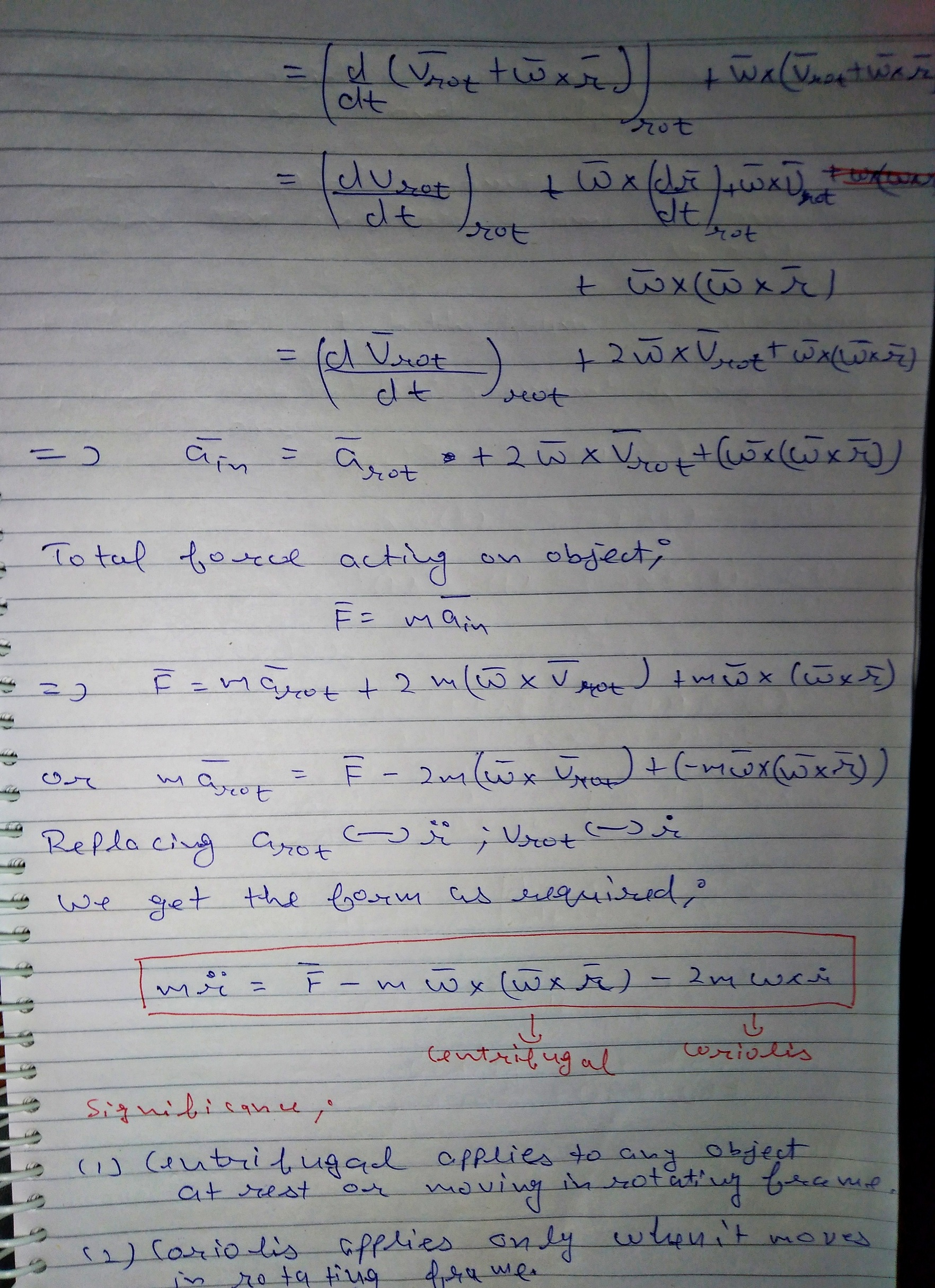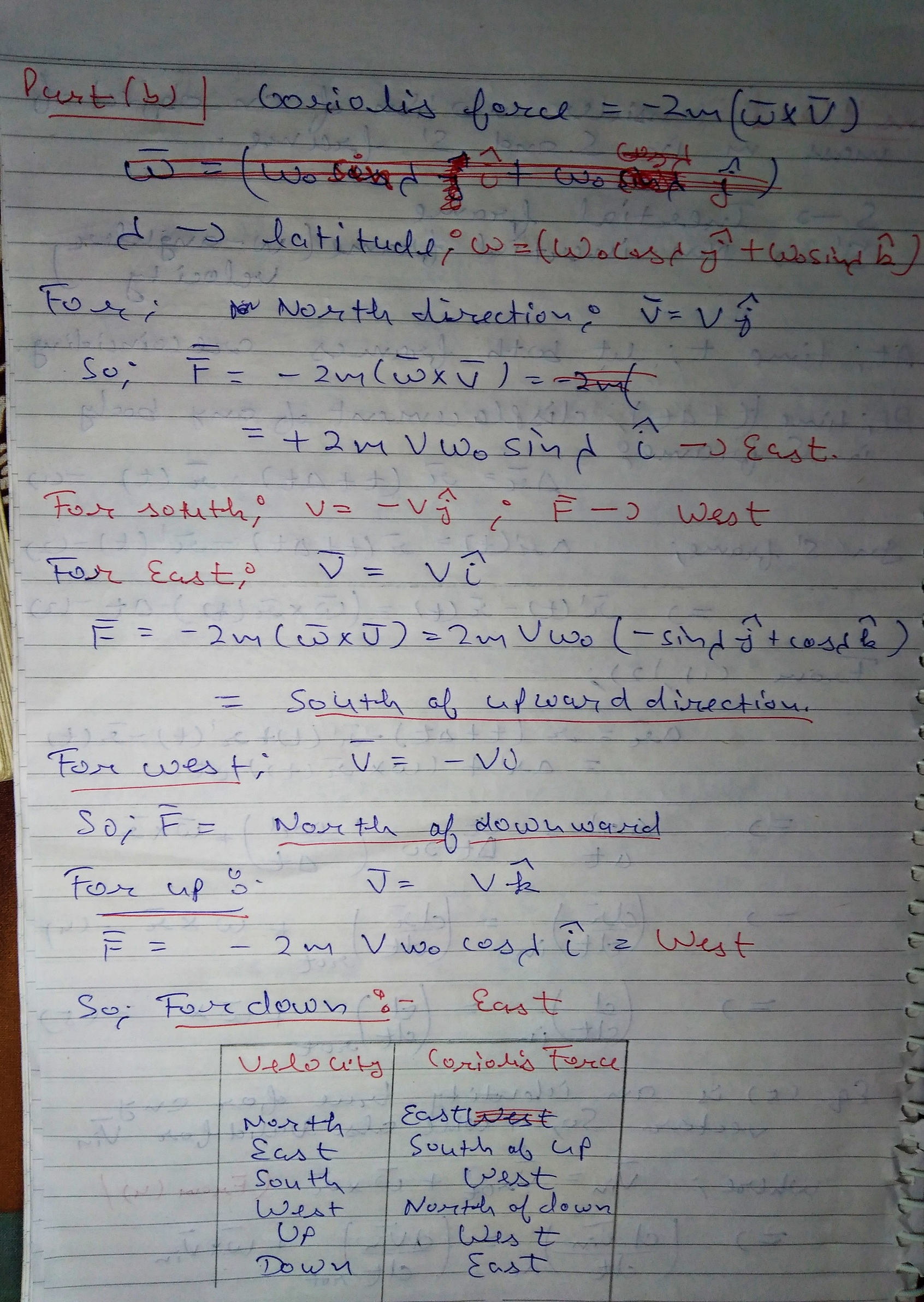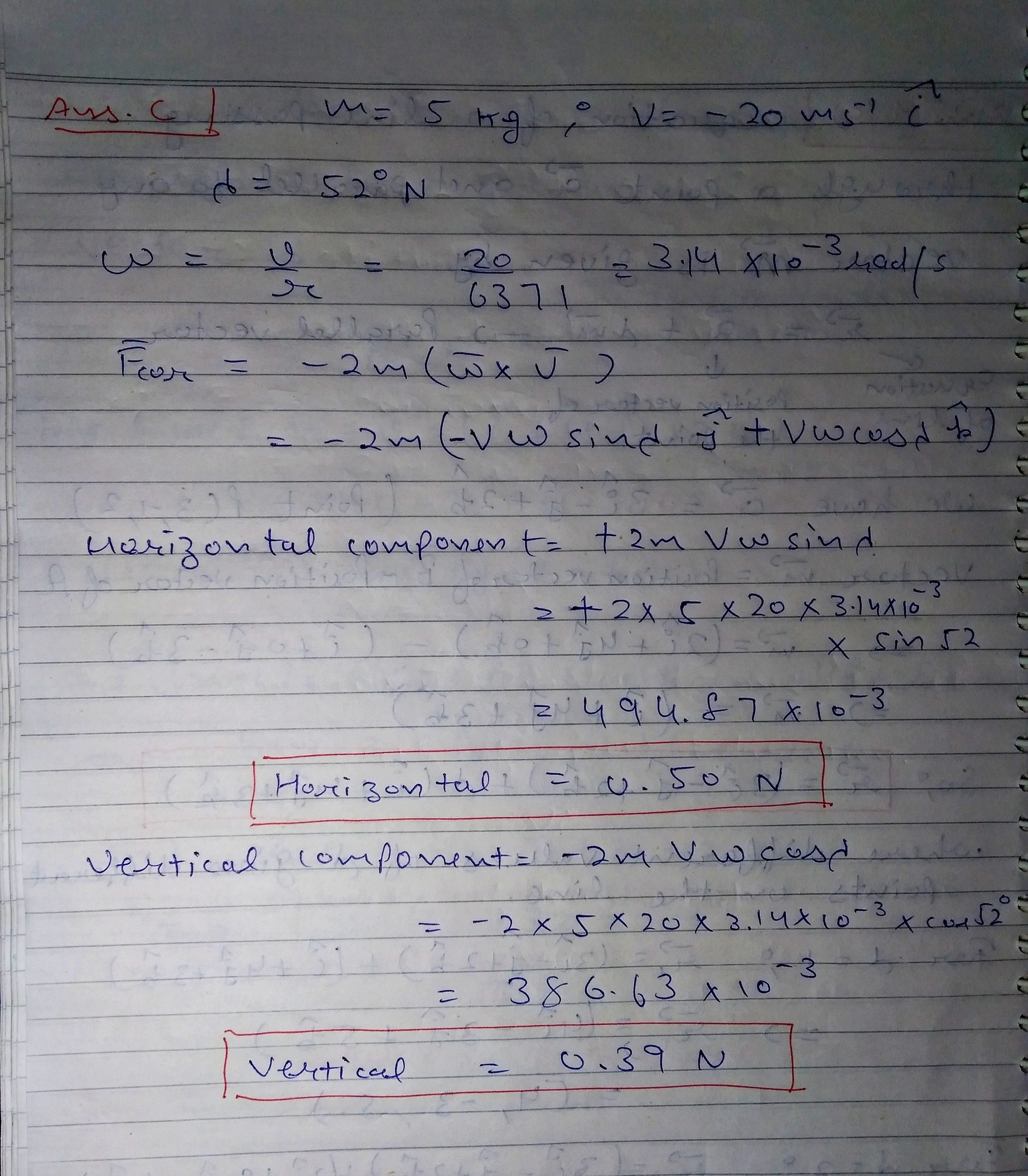#### Earn Coin

Coins can be redeemed for fabulous gifts.

Similar Homework Help Questions
• ### QUESTION 4. (4a) Show that mi DF - 2mw xi – mü x (w x M)...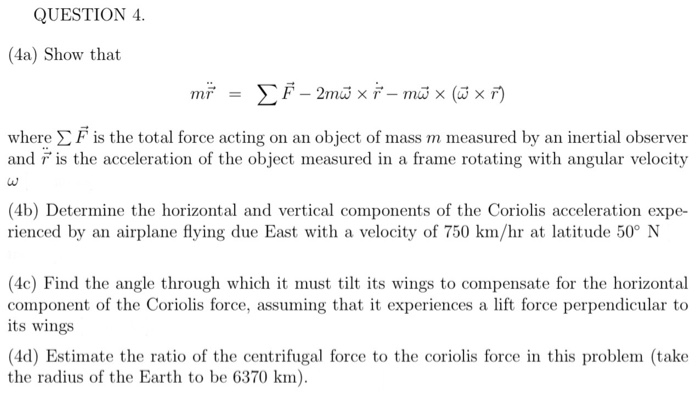QUESTION 4. (4a) Show that mi DF - 2mw xi – mü x (w x M) where is the total force acting on an object of mass m measured by an inertial observer and is the acceleration of the object measured in a frame rotating with angular velocity (4b) Determine the horizontal and vertical components of the Coriolis acceleration expe- rienced by an airplane flying due East with a velocity of 750 km/hr at latitude 50° N (4c) Find the...

• ### QUESTION 3. (3a) Show that - 2mü xi – mū x (W x ) where F...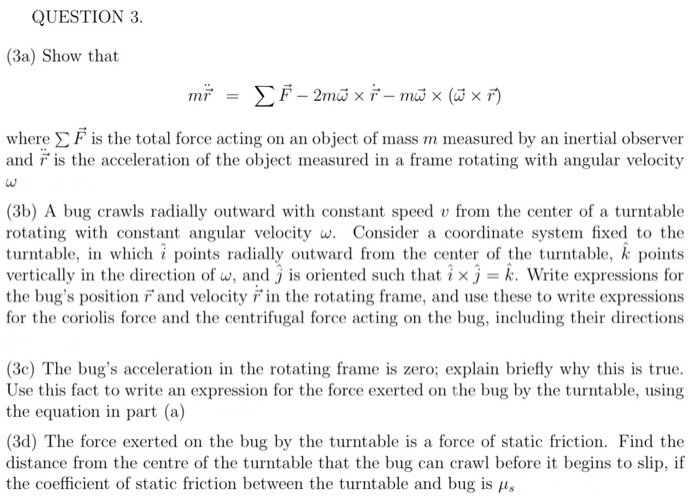QUESTION 3. (3a) Show that - 2mü xi – mū x (W x ) where F is the total force acting on an object of mass m measured by an inertial observer and is the acceleration of the object measured in a frame rotating with angular velocity الها (3b) A bug crawls radially outward with constant speed v from the center of a turntable rotating with constant angular velocity w. Consider a coordinate system fixed to the turntable, in which...

• ### Question 2 According to Newton's Laws of Motion, if I throw a baseball in outer space...

Question 2 According to Newton's Laws of Motion, if I throw a baseball in outer space - far from any other object- how will that ball move over time? (For the sake of this problem, let's neglect my mass) After the force of my hand no longer acts on the ball: Question 2 options: the ball will move forward, but it will gradually slow down and come to a stop once the force of my hand no longer acts on...

Free Homework App